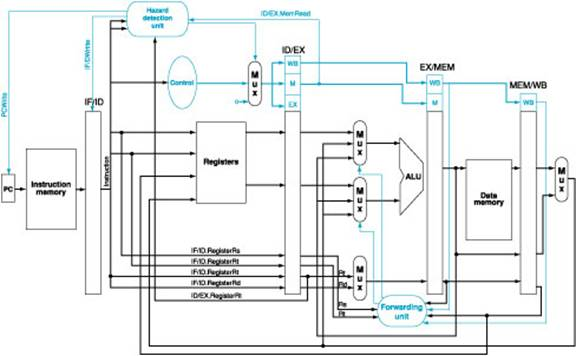Computer Organization and Structure

Homework #5

Due: 2005/1/4

1.      Identify all of the data dependencies in the following code. Which dependencies are data hazards that will be resolved via forwarding? Which dependencies are data hazards are will cause a stall?

lw    \$6, 200(\$3)

2.      Consider executing the following code on the following pipelined datapath:At the end of the fifth cycle of execution, which registers are being read and which register will be written? In addition, explain what the forwarding and the hazard detection units are doing during the fifth cycle. If any comparisons are being made, mention them. Furthermore, list all the inputs and outputs of the forwarding unit of the above figure. Give the names, the number of bits, and brief usage for each input and output.

3.      We have a program core consisting of five conditional branches. The program core will be executed thousands of times. Below are the outcomes of each branch for one execution of the program core (T for taken, N for not taken).

Branch 1: T-T-T

Branch 2: N-N-N-N

Branch 3: T-N-T-N-T-N

Branch 4: T-T-T-N-T

Branch 5: T-T-N-T-T-N-T

Assume the behavior of each branch remains the same for each program core execution. For dynamic schemes, assume each branch has its own prediction buffer and each buffer initialized to the same state before each execution. List the predictions for the following branch prediction schemes:

a.       Always taken

b.      Always not taken

c.       1-bit predictor, initialized to predict taken

d.      2-bit predictor, initialized to weakly predict taken

What are the prediction accuracies?